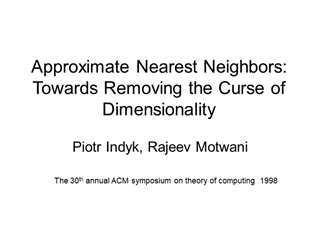# Approximate Nearest Neighbors Towards Removing the …

0

#### Presentation Transcript

• 1.Approximate Nearest Neighbors: Towards Removing the Curse of Dimensionality Piotr Indyk, Rajeev Motwani The 30th annual ACM symposium on theory of computing 1998
• 2.Problems Nearest neighbor (NN) problem: Given a set of n points P={p1, …, pn} in some metric space X, preprocess P so as to efficiently answer queries which require finding the point in P closest to a query point qX. Approximate nearest neighbor (ANN) problem: Find a point pP that is an –approximate nearest neighbor of the query q in that for all p'P, d(p,q)(1+)d(p',q).
• 3.Motivation The nearest neighbors problem is of major importance to a variety of applications, usually involving similarity searching. Data compression Databases and data mining Information retrieval Image and video databases Machine learning Pattern recognition Statistics and data analysis Curse of dimensionality The curse of dimensionality is a term coined by Richard Bellman to describe the problem caused by the exponential increase in volume associated with adding extra dimensions to a (mathematical) space.
• 4.Overview of results and techniques These results are obtained by reducing -NNS to a new problem: point location in equal balls.
• 5.nearest neighbor search (NNS) -nearest neighbor search (NNS) Ring-Cover Trees Point location in equal balls (PLEB) - Point location in equal balls (PLEB) Locality-Sensitive Hashing Proposition 1 Proposition 2 The Bucketing method Proposition 3 Random projections Content
• 6.Definitions
• 7.Theorems
• 8.Constructing Ring-cover trees
• 9.Analysis of Ring-cover trees
• 10.Definitions
• 11.Locality-Sensitive Hashing
• 12.The Bucketing method We decompose each ball into a bounded number of cells and store them in a dictionary. The bucketing algorithm works for any lp norm.
• 13.J. L. Lemma